# Hardest colleges to get into in Kentucky

Top 10 colleges in Kentucky with the lowest acceptance rates
What are the hardest colleges to get into in Kentucky? We've got you covered. We've compiled a national college database and have created a list of the hardest universities to get into in Kentucky below. These are the hardest 4 year colleges to get into in Kentucky, and sometimes some of the hardest colleges to get into in the US. You could even say these are the best colleges in Kentucky. We also include each college's average SAT and ACT scores so that you can see where you're most competitive. Read on to find out more.

## Berea College acceptance rate

Berea College acceptance rate is 38.2%.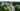The average SAT score for Berea College is 1123.

• SAT composite: 1123
• SAT math: 570

The average ACT score for Berea College is 25.

## Kentucky Christian University acceptance rate

Kentucky Christian University acceptance rate is 40%.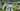The average SAT score for Kentucky Christian University is 1030.

• SAT composite: 1030
• SAT math: 520

The average ACT score for Kentucky Christian University is 20.

## Kentucky State University acceptance rate

Kentucky State University acceptance rate is 45.4%.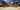The average SAT score for Kentucky State University is 950.

• SAT composite: 950
• SAT math: 470

The average ACT score for Kentucky State University is 19.

## Midway College acceptance rate

Midway College acceptance rate is 56.7%.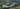The average SAT score for Midway College is 1050.

• SAT composite: 1050
• SAT math: 520

The average ACT score for Midway College is 20.

## Baker University acceptance rate

Baker University acceptance rate is 67.3%.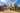The average SAT score for Baker University is 1110.

• SAT composite: 1110
• SAT math: 570

The average ACT score for Baker University is 23.

## Georgetown College acceptance rate

Georgetown College acceptance rate is 67.3%.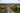The average SAT score for Georgetown College is 1090.

• SAT composite: 1090
• SAT math: 540

The average ACT score for Georgetown College is 23.

## Asbury University acceptance rate

Asbury University acceptance rate is 70.3%.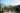The average SAT score for Asbury University is 1135.

• SAT composite: 1135
• SAT math: 550

The average ACT score for Asbury University is 25.

## Eastern Kentucky University acceptance rate

Eastern Kentucky University acceptance rate is 70.6%.The average SAT score for Eastern Kentucky University is 1040.

• SAT composite: 1040
• SAT math: 510

The average ACT score for Eastern Kentucky University is 22.

## University of the Cumberlands acceptance rate

University of the Cumberlands acceptance rate is 71.2%.The average SAT score for University of the Cumberlands is 1080.

• SAT composite: 1080
• SAT math: 540

The average ACT score for University of the Cumberlands is 22.

## The University of Louisville acceptance rate

The University of Louisville acceptance rate is 72.9%.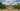The average SAT score for The University of Louisville is 1190.

• SAT composite: 1190
• SAT math: 590